International Journal of High Energy Physics
Volume 2, Issue 4-1, August 2015, Pages: 112-118

Mutual Proof of Cosmic Medium and Hubble Law

Chen Dayou

Institute of Materials and Condensed State Physics under Northwest University, Shaanxi Province, China

Email address: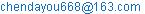To cite this article:

Chen Dayou. Mutual Proof of Cosmic Medium and Hubble Law. International Journal of High Energy Physics. Special Issue: Symmetries in Relativity, Quantum Theory, and Unified Theories. Vol. 2, No. 4-1, 2015, pp. 112-118. doi: 10.11648/j.ijhep.s.2015020401.19

Abstract: This paper proposes that the cosmic space is microscopically of granular lattice structure and the granular meta-space is called positive hole. The cosmic space which is microscopically of granular lattice structure is called quantum space. It is similar to elastic ether. We suppose the hole and the cosmic space consisting of holes are characteristic of physical elastic deformation. By introducing the constant of nuclear density and establishing the wave equation of static gravitation field, we have, for the first time, obtained the elasticity mould k of the quantum space and the mass density ρ. This paper proposes the universal concept of volume energy. Based on this, this thesis attempts to prove, in accordance with the new discoveries in the field of modern cosmology and the identity principle between space and matter, that the Hubble Law is the direct evidence for the existence of cosmic medium. In reverse, by analyzing the Hubble Law in light of dynamics according to the physical features of cosmic medium, we set forth the argument that the existence of cosmic medium and the Hubble Law support each other.

Keywords: Meta-Space, Hole, Cosmic Medium, Quantum Space, Volume Energy, Hubble Law

1. Preface

Early in the last century when no result came from the test of the light medium ether, A Einstein advanced the permanent principle of light velocity and the relativity principle, thus establishing the special theory of relativity. Today, the special theory of relativity which has already permeated into all fields of physics and the quantum mechanics constitute the two cornerstones of modern theoretic physics. It has had profound influence on the theoretic physics.

However, any theory, even the greatest theory in history is characteristic of falling into different phases. The special theory of relativity is no exception. Its deficiency lies neither in the concrete conclusions from the permanent principle of light velocity and the special theory of relativity nor in "the superstructure" of theory, but in the basis and in the fact that the special theory of relativity abandons the light medium ether, thus building the theory on the basis of vacant space. Since the physical structure of space is the basis of all natural sciences including physics and since the light medium ether is essential for theoretic physics, Einstein′s viewpoint of abandoning light medium ether has been extensively questioned.1

In the middle of the last century the establishment of modern cosmology and the discovery of the micro-wave background radiation in 3K cosmos offered an opportunity for the probe into the cosmos medium and light ether. The characteristics of the cosmos background radiation indicate that there exists only one reference system which keeps isotropic with the background radiation. Any other inertial systems which move in relation to it show the characteristic that the radiation temperature changes with the moving direction. This provides a basis for setting up an absolute and static reference system. Faced with the experimental fact of micro -wave background radiation, P.A.M. Dirac said: "We may say that the cosmic background radiation is symmetrical only for one observer. This preferential observer is static in some absolute sense. Perhaps, he is static to ether. This exactly runs counter to Einstein′s viewpoint."2

The author of this thesis is an advocator of cosmos medium existence and a propagator of the medium transmission of light and electro-magnetic radiation. But here "ether" is no longer used to name the cosmic medium. His basic view is such that the cosmic space is microscopically of granular structure and consists of meta-space; between meta-space is a lattice structure; the meta-space and the cosmic space made up of meta-space are physically characteristic of elastic deformation. This meta-space which we cannot fully understand at the moment and which possesses physical features is called hole. The cosmic space made up of holes which has the feature of elastic deformation is called elastic quantum space, quantum space for short. This thesis comes to the conclusion, under the principle of space physical identity, that the cosmic medium and Hubble Law prove each other.

2. The Elastic Deformation Constant k of Quantum Space

The quantum space is made up of holes, as is shown in Fig.(1-1). In the figure, the small circles stand for holes and the connecting lines indicate the links between them. The quantum space is physically characteristic of elastic deformation.

The Faraday field is the view of the elastic deformation of the cosmic medium. The essence of the field is the local deformation of the quantum space. Particles have definite geometric product. They exist in quantum space and exercise repelling effect on quantum space and its components, thus resulting in deformation of neighboring quantum spaces. The gravitation field of particles refers to one that is stimulated by the force of their own geometric mass repelling quantum spaces. It is characteristic of isotropy.

Nuclear physics reveals that nuclear density is a constant (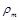). The nuclear radius and the cubic root of quantity A have definite linear relationship. Their relationship formula is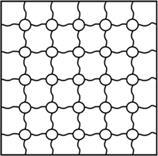Fig. (1-1). A Sketch of Quantum Space.

The small circles stand for holes. The connecting lines refer to the action between holes. The cosmic space is of granular lattice structure.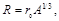(1-1)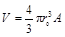.                                        (1-2)

The nucleus is approximately a sphere and in the formula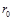is a constant (=1.20~1.22 fm).The formula(1-2) shows that nuclear matter is even. Different nuclei have the same mass density which is indicated by. If=1.21(fm), then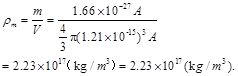3

The constant feature of nuclear density has special implication. It tells us that any objects, so long as they have the same mass, have the same geometric volume if their atomic nuclei and extra-nuclear electrons are pressed close to each other. Their effect on the quantum space can be expressed with their post-press geometric volume. When we consider the geometric volumes which particles and heavy things occupy in quantum space, we always believe they have mass density. Or we understand it as the accumulation of geometric volumes of all nucleons and extra-nuclear electrons of heavy things.

We suppose the quantum space is of elastic deformation feature and the extremely elastic quantum space and holes do not absorb the function transmitted by it. Therefore, on any concentric sphere surface with particle barycenter as its heart and embracing particles, the repelling function transmitted by holes is a constant which is in direct proportion to geometric volumes of particles. It is indicated with kV with k being a proportional constant.

On the other hand, particles are effected by the restoration function of quantum space tending a balanced state. This function is imposed on particles by holes. It is one of the two (positive and negative) sides of the same function, the other being the repelling function of particles on quantum space. In quantity, they are the same constant kV.

E is used to indicate the strength of restoration function of quantum space and M is used to refer to the strength of repelling function of particles on quantum space. So for any space of particle neighborhood, we always get

M= -E                                (1-3)

And also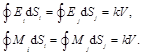(1-4)

On the sphere surface with particle barycenter as its heart, E and M are equal in size, so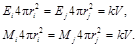(1-5)

Please note the constant feature of. Even if we give no consideration to its density significance, we can always use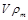to define a new physical quantity and the repelling function of equivalent and characterization particles on quantum space. We call this new physical quantity mass and indicate it with m. If we substitutem for V in formula(1-5), then we get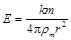(1-6)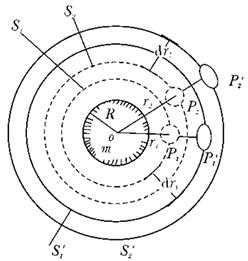Fig. (1-2). A Sketch of Radiation Displacement of Holes.

pared with S1 and S2 shells,the increments of enclosed volumes of S1′ and S2′ are both V.

The figure (1-6) is the expression formula of the gravitation field of particles. It now has a new meaning, i.e. the strength of quantum space′s restoration of balanced state at the particle′s barycenter distance r.

The particle′s repelling function on quantum space is shown in Fig.(1-2). In the free space there is now a particle with its barycenter at O, its mass being m, its radius being R and its volume being V. Influenced by particles′ repelling force, the holes which were formerly distributed on the shells of spheres S1 and S2 are removed respectively to the shells of spheres S1 and S2. Suppose holes are extremely elastic, they dont change in volume during the process of deformation and removal, or the deformation quantity is high-grade minimum. Now, both S1 and S2 sphere shells embrace holes with V volume. So, compared with the former S1 and S2, their volume increases are both volume V of particles.

The hole P1 on the former S1 shell shifts to P1 on S1′ shell and the radial displacement is P1P1=dr1. The hole P2 on the former S2 shell shifts to P2 on S2 shell. The radial displacement is P2P2′=dr2. The volume increases of S1 and S2′ parcels are both V. So we have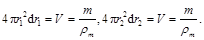Generally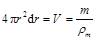(1-7)

So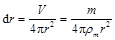(1-8)

Compare Formula(1-8) with Formula(1-6) and we immediately get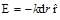.                           (1-9)

Formula(1-9) shows that the gravitation field strength is in direct proportion to the radiation displacement dr of holes in this place and shares the same form as R. Hooke Law. The k in the formula is the elastic deformation constant of quantum space. From the above analysis, we know that k really has the same dimension as the elastic deformation coefficient. The value of k may be given, with the gravitation being the constant G, by the following formula.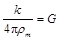(1-10)

If the numerical values of the gravitation constant G(G=6.67×10-11N·m2/kg2) and the nuclear densityare put into the formula, we have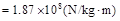k is the elastic deformation constant of quantum space and the first characteristic quantity of quantum space. Here we may give the following principle about the origin of mass: in quantum space if the volume occupied by the hole is V and the repelling effect on quantum space is kV, the hole mass is defined as.

3. The Fluctuation Equation of Fixed Gravitation Field and the Mass Density ρ of Quantum Space

Holes and quantum space have elastic deformation restoration ability, which is the sole physical property we give to holes and quantum space. The quantum space consists of separate holes and micro-particles move inside the quantum space. Every and each change of space position has to overcome the binding force of quantum space and thus repels the neighboring holes on the one hand, and on the other hand it is effected by quantum space restoring its balance. Since the geometric sense of particles is sufficiently insignificant, the movement of micro-particles is intrinsically characteristic of disconnection in this granular and eladtic quantum space.

As is shown in Fig.(2-1),let′s suppose S and S′ are neigbboring hole layers and their separation is the hole diameter d0. We hold the view that the movement of particles from S to S′ is characteristic of hops. That is to say, particles have a short stay in any of the hole layers. Once the restoration effect of quantum space on particles reaches a given value, they hop from S to S′. In other words, particles move intermittently. The interruptedness, or discontinuity, of micro-particle movement is determined by the elastic and granular space structure. Further analyses prove that the discontinuous feature of particle movement is the basis of quantization of energy and action in the microscopial field.4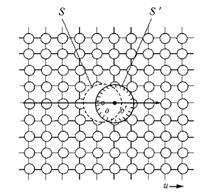Fig. (2-1). A Sketeh of the Discontinuity of Micro-particle Movement.

The discontinuous feature of particle movement is determined by the granular and elastic space structure.

If particles hop in an instant at a certain point in quantum space, the repelled neighboring space with particle barycenter as its heart restores to the balanced state. For space points with different barycenter distances, this process is not isochronic. Those holes which have not yet restored to the balanced state will exercise force on those which have restored and cause them to depart from the balanced state again. This causes the quantum space of particle′s neighboring field — the gravitation field stimulated by particles — to fluctuate.

The fluctuation of fixed gravitation field stimulated by the transient jump of particles transmits isotropically with the particle barycenter as the heart. From the unitarity, we know that the fixed gravitation wave is characteristic of spherical longitudinal wave. The meaning of fixed here refers to the fluctuation of fixed gravitation field with a certain fixed point as the heart. To derive the equation for the fluctuation of fixed gravitation field, we choose a hole pillar a-b at the particle barycenter distance r, as is shown in Fig. (2-2)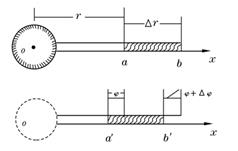Fig. (2-2). A Sketch of Wave Fluctuation of Fixed Gravitation Field.

The fixed gravitation wave is characteristic of spherical longitudinal wave. The strength of dynamic field is in direct proportion to pillar′s relative variant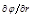.

Suppose the pillar′s sectional area is ΔS, its length is Δr and E stands for the strength of gravitation field. So the difference of gravitation field strength at the two ends a-b of the pillar is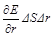Suppose the mass density of quantum space is ρ, the mass of pillar a-b is ρΔSΔr. Particles jump instantaneously and the strength difference of the two gravitation fields at the ends cause the pillar to vibrate. If its vibration velocity is u, the second law of Newton mechanics is applied to the hole pillar. So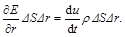.                            (2-1)

From Fig.(2-2) we know that the amplitude of pillar isat a and+Δat b. For such longitudinal fluctuation of the static gravitation field, the amplitude difference Δis the changing amount of the pillar′s length a-b.and dare the functions of r and t. When the Δt has sufficient hours,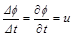,                              (2-2)

So, Formula (2-1) may be re-written as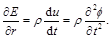(2-3)

During the fluctuation of gravitation field, the characteristics of static field strength E no longer exist, but the basic relationship shown in Formula (1-9) still exists between the field strength and the longitudinal displacement of holes in this place. In the fluctuation, the dynamic field strength is indicated by the longitudinal relative shift of pillar a-b. when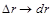,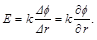(2-4)

Further,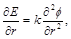(2-5)

Compare Formula (2-3) with Formula (2-5), we get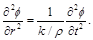(2-6)

On the other hand, we know from the standard equation of spherical waves that amplitude isin direct proportion to r. So amplitudemay be expressed with the following formula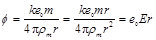,                  (2-7)

In the formula, E stands for the gravitation field strength and e0 is the amplitude proportional constant. Substitute Formula (2-7) into Formula (2-6), we get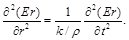(2-8)

Formula (2-8) is the fluctuation equation of gravitation field. In the formula, k/ρ is the square of phase velocity of gravitation wave. The gravitation wave and the electro-magnetic wave are both transmitted by hole vibration. They have the same velocity, i.e.: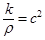,                                         (2-9)

Substitute the values of k and c into the formula, we get the mass density ρ(ρ=2.08×10-9kg/m3) of quantum space (the cosmic space). ρ stands for the second characteristic amount of quantum space and light velocity c is the 3rd characteristic amount. The three characteristic amounts are linked by Formula (2-9).5

4. Identity of Space and Matter

The mechanics of relativity theory gives the function of particles.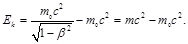.                (3-1)

The above formula may be re-written into the following according to L. de Broglie relation formula.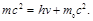(3-2)

In the formula, h is the M. Planck constant and v is the de Broglie wave frequency of moving particles. The stress of mass-energy relation formula is the interpretation of static energy. The understanding of static energy can help us recognize the identical relationship between matter and space.

The cosmic space is a quantized elastic space. Particles occupy the quantum space with their own geometric volume, making neighboring quantum space deform. The subordinate surface has the potential of elastic deformation in correspondence to the deformation quantity. This energy is in direct proportion to the particle volume V. In quantity, it is equal to the repelling force kV of particles on quantum space. So the energy is called the volume energy of particles, i·e· the energy of particles for occupying the volume of quantum space. If it is expressed by EV, then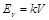.                                       (3-3)

k is the elastic deformation constant of space. In the field of nucleus and subnucleus particles, the volume energy is already proven by experiments.6 Here we hold that volume energy is of universal significance. Any object has the energy corresponding to its own volume. However, this volume is not in its normal sense. It refers to the volume an object occupies the quantum space. If the mass of the object is m, its volume cnergy is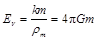.                    (3-4)

In the formula G is the gravitation constant. A kilogram matter has the volume energy of 8.38×10-10(J).

Spin is an intrinsic attribute of particles. On the one hand, spin separates particles from quantum space and forms an infinitesimal sphere which may drift in the quantum space, i.e. matter particles. On the other hand, spin enables particles to shield matter infinitesimal of strong bound state inside themselves. This infinitesimal is the hole that appears curly. Once the spinning state of particles is destroyed, particles disintegrate immediately, spraying out holes while generating new particles. Holes restore their free state from curly state in their flying process. They unfold to become components of quantum space. That is to say, space and matter have identity and homology. The so-called static energyof particles refers to the volume energy of particles with static volume of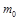. after their mass is destroyed and their volume expands.

Let′s consider an ideal state. When particles disintegrate and fall into oblivion, isotropy expands. When the holes discharged while particles disintegrate and die out are in a balanced state with quantum space, the mass density tends to change fromto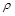and the volume changes from V to V′. At this moment, the geometric volume which expands in quantum space is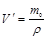.                                         (3-5)

The corresponding volume energy is kV. So the volume energy transformed due to volume expansion is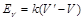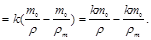(3-6)

From Formula (2-9), we have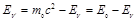.              (3-7)

Formula(3-7) shows that the energy released by matter annhilation equals the difference between static energy and volume energy. After neglecting the volume energy EV, and only after such neglect can we have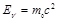.                                    (3-8)

Besides, Formula (3-6) also reveals the meaning of this important physical quantity of energy: energy is the physical quantity of ability of characteristic particles interfering in quantum space. For vibrators it is in the form of electro-magnetic radiation. For moving particles, it is in the form of de Broglie wave. For static particles it is in the form of annihilation and expansion. 7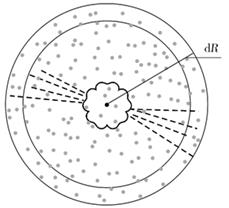Fig.( 3-1). A Sketch of Matter Annihilation Effect.

Matter annihilation ejects holes to promote the expansion of cosmic space. The grey spots indicate the newly generated holes.

Let′s take the annihilation of positive and negative electrons as example. Positive and negative electrons eject hole spouting while they collide, disintegrate and die out. The hole spoutings fly in quantum space and stop moving at a certain point when obstructed by quantum space. They unfold to become free holes. Likewise, if the lost mass due to star′s thermonuclear reaction is dM, this lost mass is naturally transformed into quantum space indicated with dM/. This promotes space′s outward expansion. The annihilation effect of mass is shown in Fig. (3-1)

5.A Dynamical Analysis of Hubble Law

Relative to galactic center by measuring the spectral red-shift of known galaxies.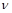=HD                                        (4-1)

Formula (4-1) is called Hubble Law. In itstands for the retrogression velocity of galaxy; D refers to the distance between known galaxies to the galactic center; H indicates Hubble constant. The significance of Hubble Law lies in the fact that astronomic observation proves the cosmic expansion. According to quantum space theory, the motive force of universe expansion comes from thermo-nuclear reaction of fixed stars. Under limited hypothetical conditions, the mass-energy relation formula of Einstein can give a dynamic explanation to universe expansion.

The matter mass releases holes when being annihilated. Its mass density tends to becomefrom.Its geometric volume swiftly expands. Therefore, the speed of cosmic expansion depends on the mass loss in the thermo nuclear reaction of fixed stars. Suppose the total mass of fixed stars in the universe is M and the mass loss of fixed stars in their thermo nuclear reaction within time interval dt is dM. Generally, dM is directly proportional to total mass M and also to reaction time dt. If we make λ a proportional constant, then

dM=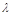Mdt                                (4-2)

Suppose the universe is a sphere and inter-stellar matter is evenly distributed, and the radius corresponding to to time is R0. Then, the cosmic geometric expansion satisfies the following relationship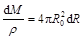(4-3)

If Formula(4-2) is put into Formula (4-3), then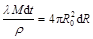(4-4)

After being sorted out, the cosmic expansion velocity is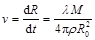(4-5)

The two sides of Formula (4-4) are multiplied by constant k, which shows the potential energy of quantum space expansion, transformed from the mass loss of fixed stars. The potential energy equals, in quantity, the energy dMc2 of thermo radiation of fixed stars. Formula (4-5) indicates the cosmic expansion velocity V which is in direct proportion to the total mass M of fixed stars, but is in invert proportion to the radius R of the universe.

The universe is even on a large scale. For any observed sections, the mass density of fixed stars is isotropic and also remains a constant. Suppose the mass density is ρn, then Formula (4-5) becomes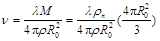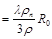(4-6)

Formula(4-6) shows that the velocity of cosmic expansion is in direct proportion to the mass density ρn of fixed stars and the product of the cosmic radius R .8The meta-space holes generated from matter annihilation evenly stay in the universe including galaxies, celestial bodies and matter, promoting cosmic expansion. But the expanding force is not big enough to overcome the electro-magnetic force between matter and the gravitation inside galaxies. Thus, the cosmic expansions seems to be the expansion of cosmic space and is characteristic of isotropy. In fact, matter annihiltion happens in radiation, making the radiation pressure become the motive force of cosmic expansion. What Hubble law aims to reveal is the law governing the moving velocity of different galaxies in the same observed section. At this time ρn is isotropic and remains a constant .This is similar to claiming that the cosmic sphere is expanding at a speed directly proportional to its own dimension. Any two galaxies in the sphere are retrogressing at a speed directly proportional to the distance between them and with the aid of cosmic expansion. The coefficient λρn/3ρ is the Hubble constant H0 of t0 moment. H is the function of the cosmic evolution age t.

6. Conclusion

The cosmic space, microscopically of granular lattice structure, has the physical attribute of elastic deformation. This fundamental hypothesis is not different in nature from the elastic ether theory. However, by the introduction of the nuclear density constant ρm we obtain the elastic deformation constant k and the space mass density ρ of quantum space. This indirectly proves the material attribute of the cosmic space. The matter mass is annihilated and the mass density tends to become ρ from ρm and static energy transforms into volume energy. This naturally promotes the expansion of cosmic space. Hubble law is a law of experimental observation in the astronomical field. On the other hand, cosmic expansion needs an expansion source. Without such a source the expansion is incomprehensible. The source is exactly the meta-space holes released when matter integrate and die out. That′s why we claim that the existence of cosmic medium and Hubble Law support and prove each other.

References

1. Tan Shusheng, From Special Theory of Relativity to Standard Time-Space Theory [M], Changsha: Hunan Press of Science & Technology, 2007, 212~213
2. Dirac, Why We Believe in Einstein′s Theory [J], Series on natural sciences and philosophy, 1983, (3), 13
3. Atomic Data and Nuclear Data Table[J],1974,14,479
4. Chen Dayou, On Generation and Significance of Mass [M], Xi′an : Northwest University Press, 2007, 107~113
5. John, D·Barro, translated by Bian Yulin, The origin of the Universe [M], Shanghai: Shanghai Science & Technology Press, 1995
6. Xu Sida, Nuclear Physics [M],Beijing:Qinghua University Press,1992, 53-55
7. Chen Dayou, On Generation and Significance of Mass [M], Xi′an: Northwest University Press, 2007, 111~113.
8. Malcolm. longier, translated by Wang Wenhao, The Cosmic Century [M] changsha: Hunan Science & Technology Press, 2010,380-386.

 Contents 1. 2. 3. 4. 5. 6.
Article ToolsAbstractPDF(315K)
Follow on us
PUBLICATION SERVICE
JOIN US
RESOURCES
SPECIAL SERVICES
ADDRESS
Science Publishing Group
548 FASHION AVENUE
NEW YORK, NY 10018
U.S.A.
Tel: (001)347-688-8931